Function Repository Resource:

# PrincipalAxisClustering

Quickly cluster a point cloud by recursive separation

Contributed by: Richard Hennigan (Wolfram Research)
 ResourceFunction["PrincipalAxisClustering"][{{p11,p12,…},{p21,p22,…},…}] recursively partitions the given points into approximately equal-sized clusters along their principal axis. ResourceFunction["PrincipalAxisClustering"][points,n] partitions points into at most n clusters.

## Details and Options

ResourceFunction["PrincipalAxisClustering"][points] is equivalent to ResourceFunction["PrincipalAxisClustering"][points,Automatic].
If n is a power of 2, the clusters will be approximately equal-sized.
ResourceFunction["PrincipalAxisClustering"] accepts a Method option, which decides how to separate points according to their projected values on the principal axis.
The value for Method can be one of the following:
 Median separate points into approximately equal-sized clusters Mean separate points at the center of mass

## Examples

### Basic Examples (3)

Cluster one-dimensional data:

 In:=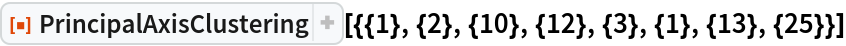Out=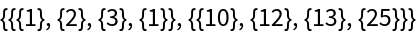Find exactly four clusters:

 In:=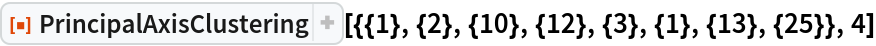Out=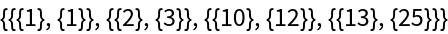Cluster vectors of real values:

 In:=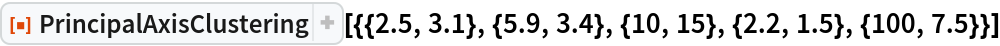Out=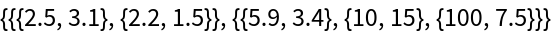### Scope (2)

Partition a 3D point cloud:

 In:=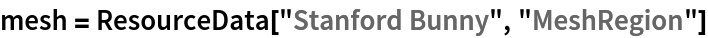Out=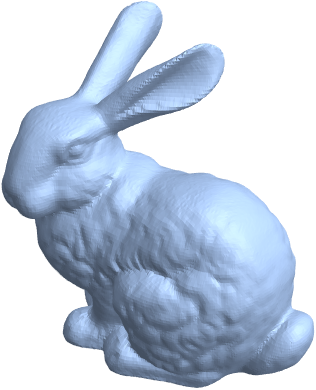In:=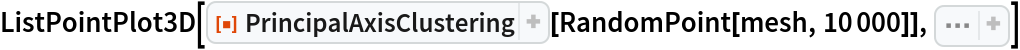Out=Cluster high-dimensional data:

 In:=Out=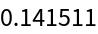### Options (3)

#### Method (3)

With the default setting of , clusters are likely to span across gaps:

 In:=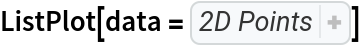Out=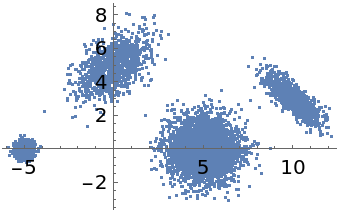In:=Out=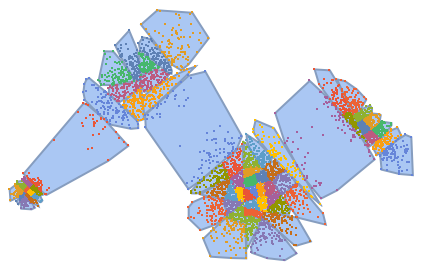Using can improve separation:

 In:=Out=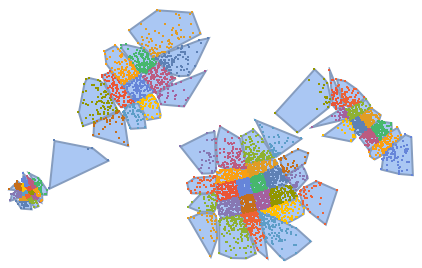However, Mean will tend to produce unbalanced cluster sizes compared to Median:

 In:=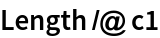Out=In:=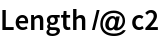Out=### Applications (2)

Downsample a large point cloud by choosing nicely spaced representative points:

 In:=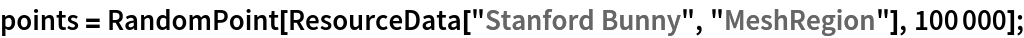In:=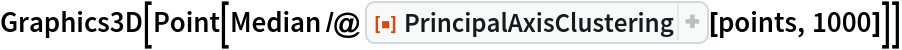Out=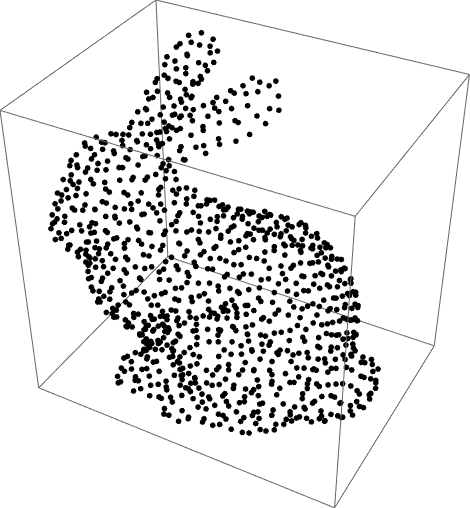Compare to random sampling:

 In:=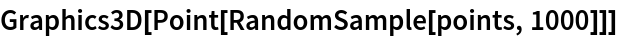Out=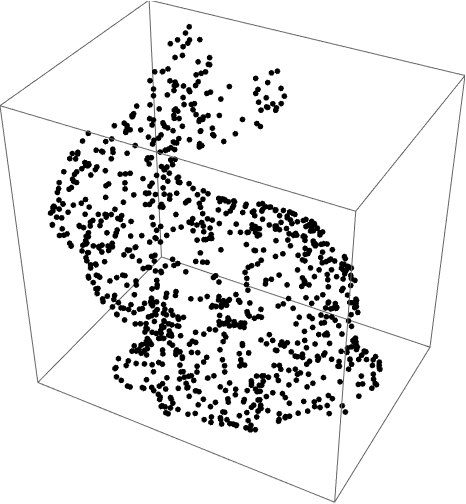### Properties and Relations (4)

The axis representing each cluster separation corresponds to the first component in PrincipalComponents:

 In:=In:=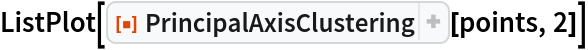Out=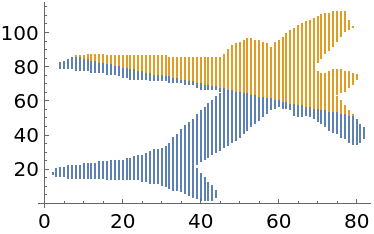In:=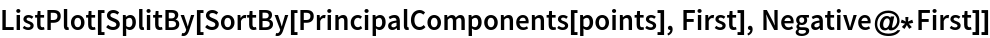Out=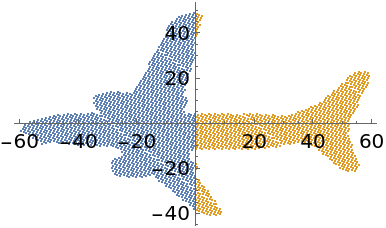The principal axis can be obtained from the Eigenvectors of the Covariance matrix:

 In:=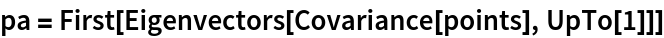Out=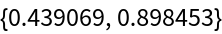Visualize the axis over the original data:

 In:=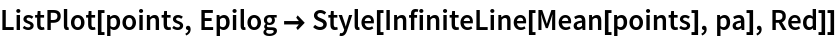Out=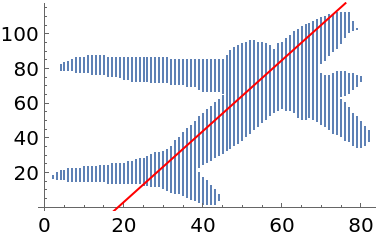Projecting the standardized points onto the principal axis gives scalar values that indicate which cluster they belong to:

 In:=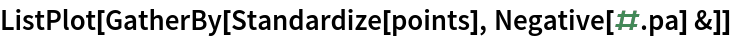Out=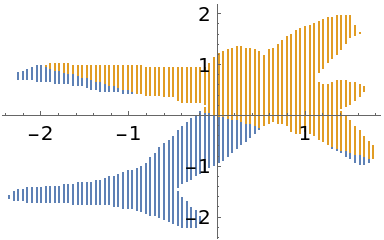PrincipalAxisClustering finds clusters very quickly:

 In:=Out=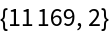In:=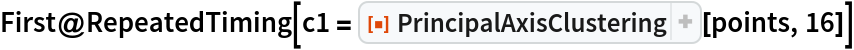Out=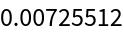Compare to FindClusters:

 In:=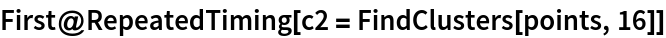Out=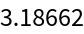PrincipalAxisClustering gives clusters that better represent the local point cloud density:

 In:=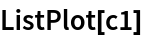Out=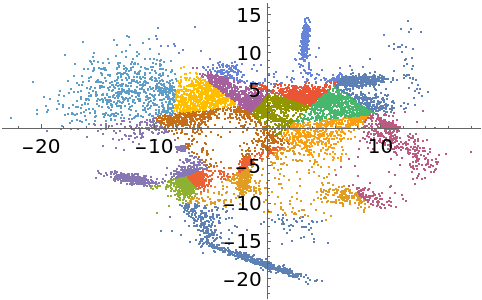FindClusters represents better separation of the data:

 In:=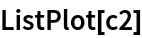Out=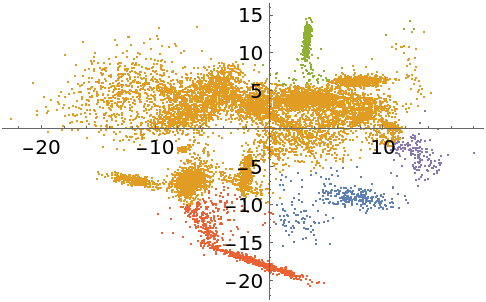PrincipalAxisClustering partitions points into non-overlapping convex regions:

 In:=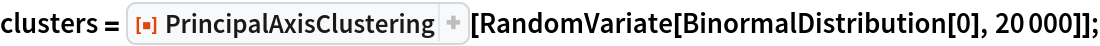In:=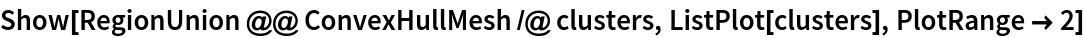Out=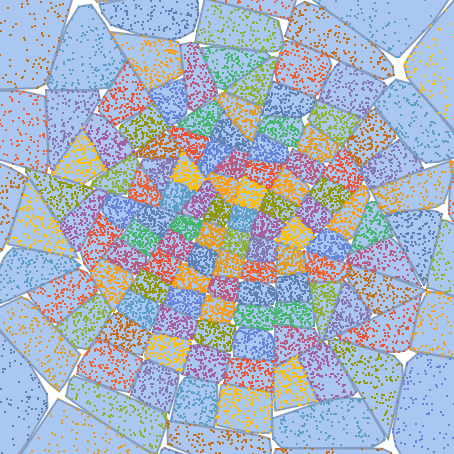The number of clusters locally scales with the point cloud density:

 In:=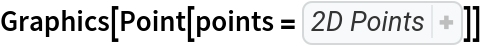Out=In:=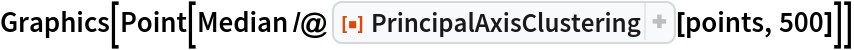Out=### Possible Issues (1)

If the number of requested clusters is not a power of 2, then cluster sizes will not be well balanced:

 In:=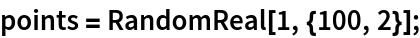In:=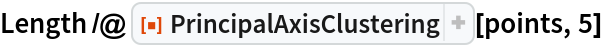Out=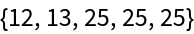In:=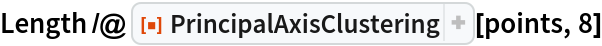Out=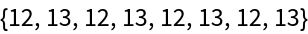### Neat Examples (2)

Visualize the recursive nature of the clustering:

 In:=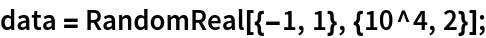In:=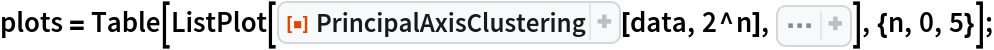In:=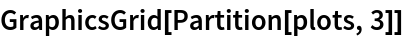Out=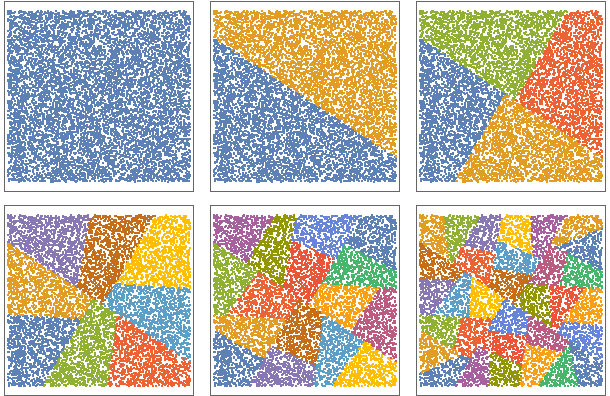Partition a point cloud into clusters:

 In:=Out=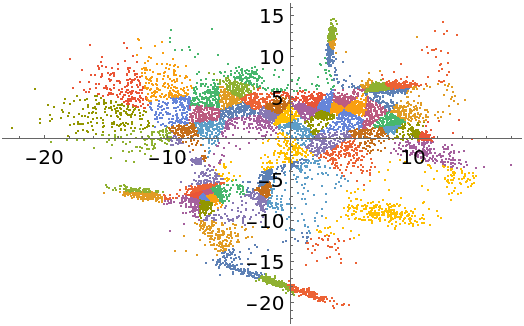Remove some outliers from each cluster:

 In:=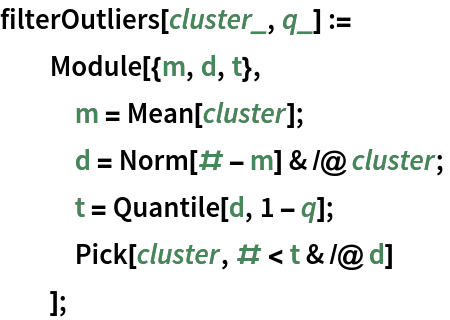In:=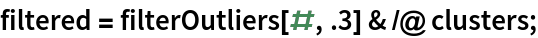In:=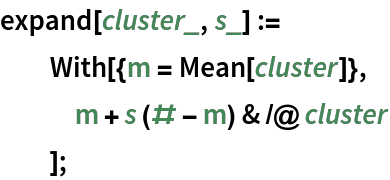Construct a parameterized topological representation of the point cloud:

 In:=Out=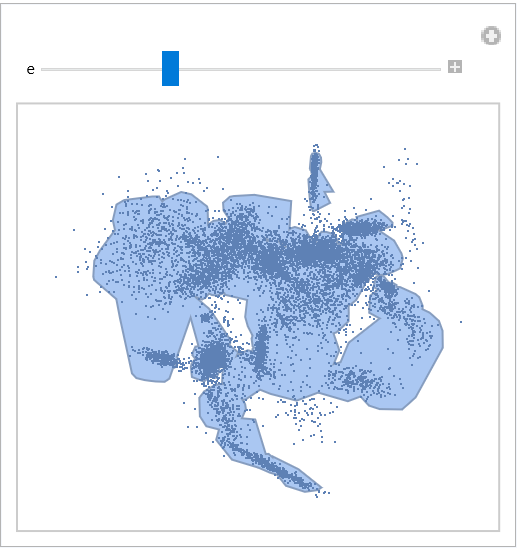## Version History

• 1.0.0 – 03 January 2022